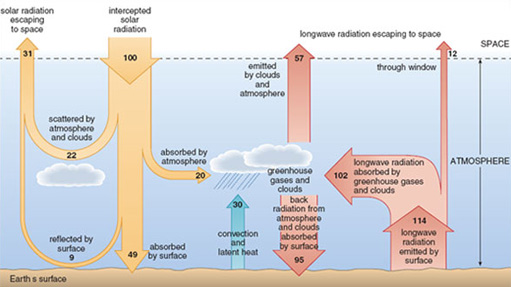Nature & Environment

### Become an OU studentClimate change

Start this free course now. Just create an account and sign in. Enrol and complete the course for a free statement of participation or digital badge if available.

# 1.4 An overview of the global energy budget

Figure 12 incorporates the additional factors considered in Section 1.3, including the non-radiative energy transfers across the surface-air boundary (green arrow). Essentially a more detailed version of Figure 7, this figure gives quantified estimates of the globally averaged energy budget for the whole Earth-atmosphere system, and its component parts. Question 3 should help you to find your way around Figure 12, and to draw together many of the key points developed so far in this chapter. Make sure to try answering it before moving on.Figure 12 Schematic representation of the overall energy budget for the Earth and its atmosphere. Figures are global annual averages expressed as a percentage of the rate per unit area at which solar radiation is intercepted by the Earth; i.e. 100 units is equivalent to 342 W m−2, as in Figure 4.

## Question 3

With reference to Figure 12:

(a) What proportion (as a percentage) of the Earth's planetary albedo is due to solar radiation reflected by the surface? Which regions of the world are likely to be mainly responsible for this contribution?

(b) Calculate the difference between the rate of energy gain and the rate of loss for: (i) the Earth's surface; (ii) the atmosphere; and (iii) the whole Earth-atmosphere system (i.e. at the top of the atmosphere). What do you conclude about the Earth's GMST?

(c) What proportion (as a percentage) of the longwave radiation emitted by the surface is absorbed by the atmosphere?

(d) Translate the 114 units of longwave radiation emitted by the surface into a rate of energy transfer (in W m−2). Explain why your answer is consistent with the fact that the Earth's GMST is higher than its effective radiating temperature (−19 °C).

(a) The planetary albedo is the proportion of incoming solar radiation reflected or (scattered) directly back to space - 31 units according to Figure 12. Surface reflection contributes 9 units or (9/31) × 100% = 29%. Snow- or ice-covered surfaces (predominantly at high latitudes) are likely to be mainly responsible, given their high albedo.

(b) (i) The total rate of energy gain by the Earth's surface is the sum of the appropriate downward-pointing arrows in Figure 2.12; i.e. (49 + 95) units = 144 units. The total loss rate is the sum of the upward-pointing arrows that originate at the Earth's surface: (30 + 114) units = 144 units. The difference is zero, so the surface is in a steady state; the GMST is not changing.

(ii) Proceeding as in (i), the total rate of energy gain by the atmosphere is: (20 + 30 + 102) units = 152 units. The total rate of loss is: (95 + 57) units = 152 units. The difference is again zero.

(iii) For the whole Earth-atmosphere system, the total rate of energy gain (solar radiation intercepted) is 100 units, and the total rate of loss is (31 + 57 + 12) units = 100 units, confirming that the whole system is also in a steady state.

(c) The proportion is (102/114) × 100% = 89% (to 2 significant figures).

(d) 100 units is equivalent to 342 W m−2, so 114 units is equivalent to (342 W m−2/100) × 114 = 390 W m−2. This is significantly higher than the rate of emission (236 W m−2; Section 1.2.1) from a body with an effective radiating temperature of −19°C. Since the rate of emission increases with increasing temperature, this implies that the Earth's GMST is higher than −19 °C.

To sum up: in Figure 12, the whole Earth-atmosphere system is in a dynamic steady state or equilibrium. Most (89%) of the outgoing longwave radiation is absorbed and recycled by the atmosphere, and ultimately re-emitted to space from higher, colder levels (Figure 9). As a result, energy circulates within the system at a higher rate than the rate of input or output at the top of the atmosphere: this is why the Earth's surface is warmer than it otherwise would be. But in a balanced state, there is no net accumulation of energy in any part of the system, and no net loss. In short, Figure 12 depicts a world where the GMST is not changing. So what might cause the Earth's GMST to change?